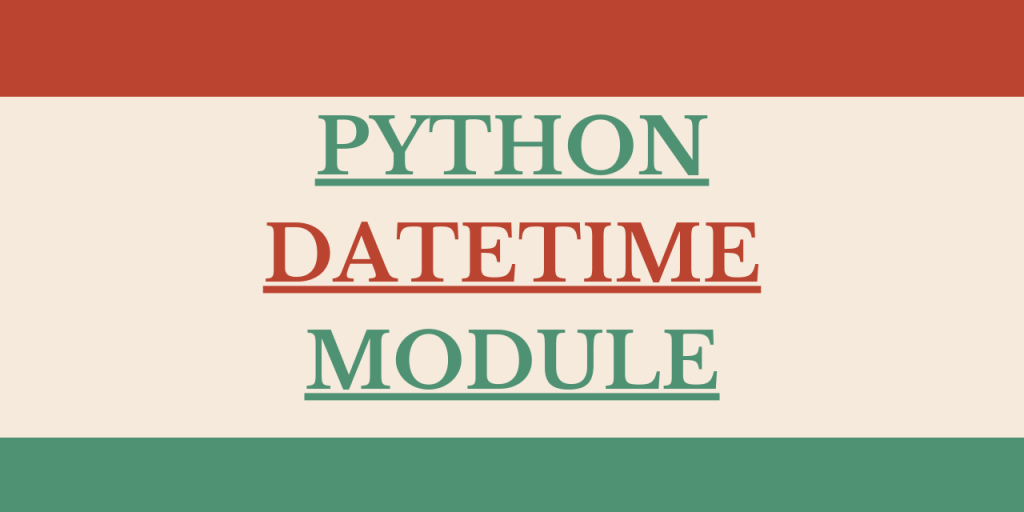# Python datetime module – An Ultimate GuideHey, folks! Hope you all are doing well. In this article, we will be focusing on Python datetime module.

This article would pay special attention to the methods or techniques to deal with the date-structure in Python. So, let us get started.

## What is Python Datetime module?

The `Python Datetime module` enables us to deal with date type data values in Python. The Datetime module consists of different classes to deal with the date and datetime expressions.

As well all know, Python does not offer any specific data type for date and time, rather, we can import and use datetime module to work with the date and time effectively.

## Classes Associated with Python Datetime module

Python Datetime module contains six (6) classes to deal with date and time values.

In Python, date and datetime expressions are actually treated as objects. Thus, these below-mentioned classes come into the picture to work with these date objects:

• date
• time
• datetime
• timedelta
• tzinfo
• timezone

Now, let us understand each of the above classes and its functionality in detail.

## 1. datetime.date class

The date class of datetime module represents the information about the date object in the date format — YYYY:MM:DD.

Syntax:

```datetime.date(year,month,day)
```

Let us understand some of the various functions offered by the Date class.

```from datetime import date

dte = date(2000,12,27)

print(dte)
```

In the above example, we have used `date() function `to convert the passed values of the day, month, and year to a standard date format.

Output:

```2000-12-27
```

In this example, we have used `date.today() function` to avail the current date from the system. Further, we have printed the present day, year, and month value as shown below:

```from datetime import date

dte = date.today()
print("Present date: ",dte)

print("Present year: ",dte.year)
print("Present month: ",dte.month)
print("Present day: ",dte.day)
```

Output:

```Present date:  2020-07-14
Present year:  2020
Present month:  7
Present day:  14
```

## 2. datetime.time class

The time class provides information specifically about the time values independent of the date expression or date objects.

We can thus access the time values in the form of standard or regularized timestamp using time class from datetime module.

Syntax:

```datetime.time(hour,min,sec)
```

Example:

```from datetime import time

tym = time(12,14,45)
print("Timestamp: ",tym)

```

Output:

```Timestamp:  12:14:45
```

In this example, we have accessed the hour, minutes and seconds value using `time() function` as shown below–

```from datetime import time

tym = time(12,14,45)
print("Hour value from the timestamp: ",tym.hour)
print("Minute value from the timestamp: ",tym.minute)
print("Second value from the timestamp: ",tym.second)
```

Output:

```Hour value from the timestamp:  12
Minute value from the timestamp:  14
Second value from the timestamp:  45
```

## 3. datetime.datetime class

The datetime class gives us information about the date as well as time values. Thus, it represents the entire date as well as time values using the functions of the class.

Syntax:

```datetime.datetime(year,month,day,hour,min,sec,microsecond)
```

In the below example, we have passed the necessary arguments and have implemented the `datetime() function` of datetime class.

```from datetime import datetime

dte_tym = datetime(2020,3,12,5,45,25,243245)
print(dte_tym)
```

Output:

```2020-03-12 05:45:25.243245
```

## 4. datetime.timedelta class

Python DateTime modules offer us the timedelta class to deal with all the kinds of date-related manipulations.

Syntax:

```datetime.timedelta(days,hours,weeks,minutes,seconds)
```

The` timedelta() function` returns a date after performing specified manipulation on the data.

```from datetime import datetime, timedelta

dte = datetime(2020,12,9)

updated_tym = dte + timedelta(days = 2,weeks=4)
print("Updated datetime: ",updated_tym)
```

In the above example, we have added days and weeks to the predefined date value.

Output:

```Updated datetime:  2021-01-08 00:00:00
```

Apart from addition, we can also backtrack the date values by subtracting particular date parts or portion of time from the datetime expression as shown below:

```from datetime import datetime, timedelta

dte = datetime(2020,12,9)

updated_tym = dte - timedelta(days = 2,weeks=4)
print("Updated datetime: ",updated_tym)
```

Output:

```Updated datetime:  2020-11-09 00:00:00
```

## Conclusion

By this we have come to the end of this topic. As seen above, datetime module plays an important role in the representation of date and time in various ways.

Feel free to comment below in case you come across any doubt.

Till then, Happy Learning!!